Courses

# Short & long Answer Question(Part-2) - Coordination Compounds Class 12 Notes | EduRev

## JEE : Short & long Answer Question(Part-2) - Coordination Compounds Class 12 Notes | EduRev

The document Short & long Answer Question(Part-2) - Coordination Compounds Class 12 Notes | EduRev is a part of the JEE Course Chemistry for JEE.
All you need of JEE at this link: JEE

Q1. Using IUPAC norms write the formula for the following: Tetrahydroxozincate(II)

Ans. [Zn(OH4)2-

Q2. Using IUPAC norms write the formula for the following: Hexaamminecobalt(III)

sulphate

Ans. [Co(NH3)6]2(SO4)3

Q3. Using IUPAC norms write the formula for the following: Pentaamminenitrito-cobalt(III)

Ans. [Co(ONO) (NH3)5]2+

Q4. Using IUPAC norms write the systematic name of the following: [Co(NH3)6]Cl3

Ans. Hexaamminecobalt(III) chloride

Q5. Using IUPAC norms write the systematic name of the following:

[Pt(NH3)2Cl(NH2CH3)]Cl

Ans. Diamminechlorido(methylamine) platinum(II) chloride

Q6. Using IUPAC norms write the systematic name of the following: c[Cr(C2O4)3]3+
Ans. Tris(ethane-1, 2-diammine) cobalt(III) ion

Q7. What is Spectro chemical series? Explain the difference between a weak field ligand and a strong field ligand.

Ans. A Spectro chemical series is the arrangement of common ligands in the increasing

order of their crystal-field splitting energy (CFSE) values.

I- < BR - < S2- < SCN- < Cl-<N3,<F-<OH-<C2O42-~H2O-~NCS-~H-<CN-<NH3<en~ SO32-<NO2-<phen<CO
Q8. [Cr(NH3)6]3+is paramagnetic while [Ni(CN)4]2−is diamagnetic. Explain why?

Ans. Cr is in the +3 oxidation state i.e., d3 configuration. Also, NHis a weak field ligand

that does not cause the pairing of the electrons in the 3d orbital. Cr3+: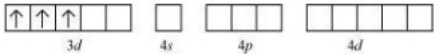Therefore, it undergoes d2sp3 hybridization and the electrons in the 3d orbitals remain

unpaired. Hence, it is paramagnetic in nature.

In [Ni(CN)4]2−, Ni exists in the +2 oxidation state i.e., d8configuration. Ni2+: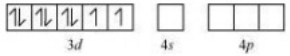CN is a strong field ligand. It causes the pairing of the 3d orbital electrons. Then, Ni2+undergoes dsp2 hybridization.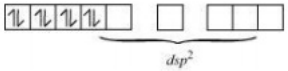Q.9 A solution of [Ni(H2O)6 ] 2+ is GREEN BUT A SOLUTION OF [Ni(CN)4 ] 2- colourless. Explain.

Ans. In [Ni(H2O)6]2+is a weak field ligand. Therefore, there are unpaired electrons

in Ni2+. In this complex, the d electrons from the lower energy level can be excited to the

higher energy level i.e., the possibility of d−d transition is present. Hence, Ni(H2O)6]2+is n coloured.
In [Ni(CN)4]2-, the electrons are all paired as CN- is a strong field ligand. Therefore, d-d transition is not possible in [Ni(CN)4]2−. Hence, it is colourless. As there are no unpaired electrons, it is diamagnetic.
Q.10  What is meant by stability of a coordination compound in solution? State the factors which govern stability of complexes.
Ans. The stability of a complex in a solution refers to the degree of association between the two species involved in a state of equilibrium. Stability can be expressed quantitatively in terms of stability constant or formation constant.
M + 3L ↔ ML3

Stability constant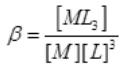For this reaction, the greater the value of the stability constant, the greater is the

proportion of ML3 in the solution.

Q1. Discuss the nature of bonding in the following coordination entities on the basis of valence bond theory:

i. [Fe(CN)6]4−

ii. [FeF6]3−

iii. [Co(C2O4)3]3−

iv. [CoF6]3−

Ans. i. [Fe(CN)6]4− In the above coordination complex, iron exists in the +II oxidation

state. Fe2+: Electronic configuration is 3d6 Orbitals of Fe2+ion: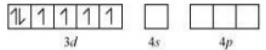As CNis a strong field ligand, it causes the pairing of the unpaired 3d electrons. Since

there are six ligands around the central metal ion, the most feasible hybridization is d2sp3. d2sp3 hybridized orbitals of Fe2+ are: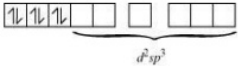6 electron pairs from CNions occupy the six hybrid d2sporbitals.Then,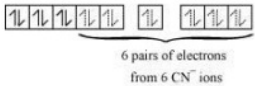Hence, the geometry of the complex is octahedral and the complex is diamagnetic (as there are no unpaired electrons).

ii. [FeF6]3− In this complex, the oxidation state of Fe is +3.

Orbitals of Fe+3 ion: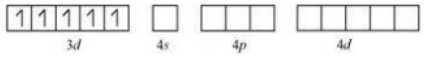There are 6 F− ions. Thus, it will undergo d2sp3 or sp3d2 hybridization. As Fis a weak

field ligand, it does not cause the pairing of the electrons in the 3d orbital. Hence, the

most feasible hybridization is sp3d2.sp3d2 hybridized orbitals of Fe are: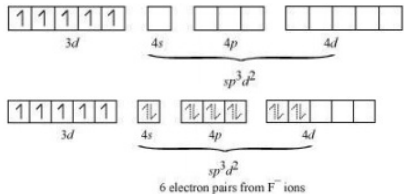Hence, the geometry of the complex is found to be octahedral.

iii. [Co(C2O4)3]3− Cobalt exists in the +3 oxidation state in the given complex. Orbitals of

Co3+ion: Oxalate is a weak field ligand. Therefore, it cannot cause the pairing of the

3d orbital electrons. As there are 6 ligands, hybridization has to be either sp3d2 or d2sp3 hybridization. sp3d2 hybridization of Co3+ :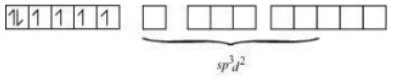The 6 electron pairs from the 3 oxalate ions (oxalate anion is a bidentate ligand)

occupy these sp3d2 orbitals.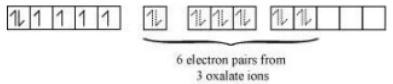Hence, the geometry of the complex is found to be octahedral.

iv. [CoF6]3− Cobalt exists in the +3 oxidation state.
Orbitals of Co3+ion: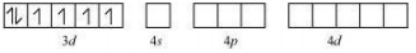Again, fluoride ion is a weak field ligand. It cannot cause the pairing of the 3d

electrons. As a result, the Co3+ion will undergo sp3d2 hybridization.sp3d2 hybridized

orbitals of Co3+ion are: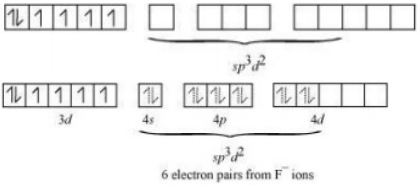Hence, the geometry of the complex is octahedral and paramagnetic.

Offer running on EduRev: Apply code STAYHOME200 to get INR 200 off on our premium plan EduRev Infinity!

## Chemistry for JEE

196 videos|450 docs|369 tests

,

,

,

,

,

,

,

,

,

,

,

,

,

,

,

,

,

,

,

,

,

;